# Object Sort Order for bwlabel, bwconncomp, and regionprops

Someone recently asked me about the order of objects found by the functions bwlabel, bwconncomp, and regionprops. In this binary image, for example, what accounts for the object order?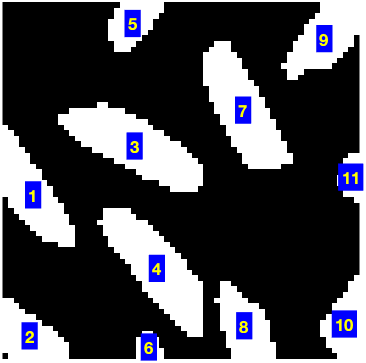The functions bwlabel and bwconncomp both scan for objects in the same direction. They look down each column, starting with the left-most column. This animation illustrates the search order: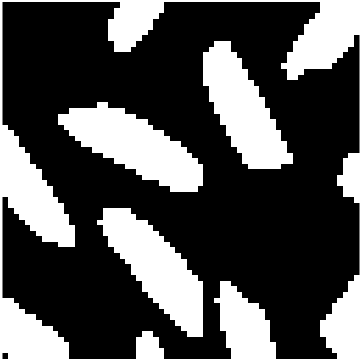Because of this search procedure, the object order depends on the top, left-most pixel in each object. Specifically, the order is a lexicographic sort of $(c,r)$ coordinates, where c and r are the column and row coordinates of the top, left-most pixel.
If you pass a binary image to regionprops, the resulting object order is the same as for bwconncomp, and that is because regionprops calls bwconncomp under the hood.
Sometimes, people who are working with images of text ask to change things so that the search proceeds along rows instead of columns. The motivation is to get the object order to be same as the order of characters on each line. (Assuming a left-to-right language, that is.) That generally doesn't work well, though. Consider this text fragment:With a row-wise search, the letter "b" would be found first, followed by "L" and then "t." This animation shows why that happens:If you want a different sort order, I recommend returning the regionprops output as a table, and then you can use sortrows. Here are a couple of examples.
url = "https://blogs.mathworks.com/steve/files/rice-bw-ul.png";
imshow(A)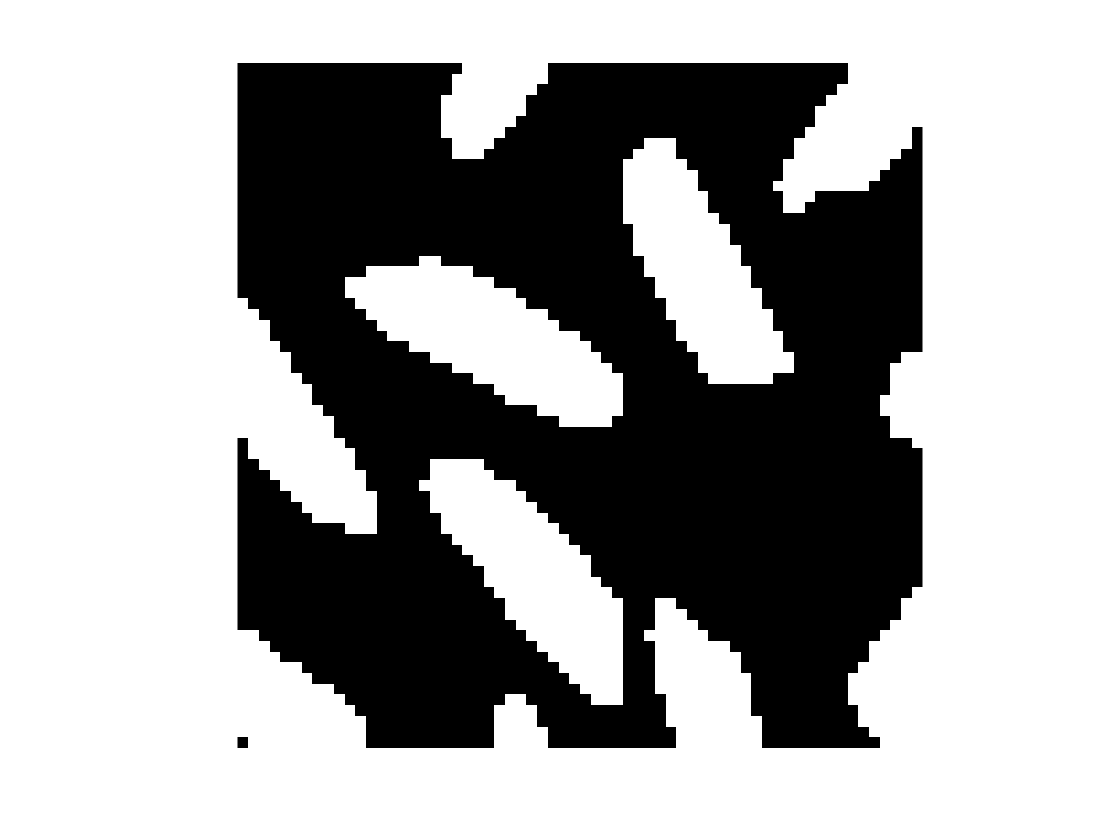props = regionprops("table",A,["Centroid" "Area"])
props = 11×2 table
AreaCentroid
11385.985535.0870
2875.367860.3563
320824.197126.3125
419928.185948.2563
56123.81974.3443
62026.750062.3500
718943.634919.8783
89743.845458.5361
911558.20877.0696
107061.785758.2286
112662.923131.8846
You could sort the objects according to their centroids, with the primary sort in the vertical direction and the secondary sort in the horizontal direction.
props.Centroid_x = props.Centroid(:,1);
props.Centroid_y = props.Centroid(:,2);
props_by_row = sortrows(props,["Centroid_y" "Centroid_x"])
props_by_row = 11×4 table
AreaCentroidCentroid_xCentroid_y
16123.81974.344323.81974.3443
211558.20877.069658.20877.0696
318943.634919.878343.634919.8783
420824.197126.312524.197126.3125
52662.923131.884662.923131.8846
61385.985535.08705.985535.0870
719928.185948.256328.185948.2563
87061.785758.228661.785758.2286
99743.845458.536143.845458.5361
10875.367860.35635.367860.3563
112026.750062.350026.750062.3500
imshow(A)
for k = 1:height(props_by_row)
x = props_by_row.Centroid_x(k);
y = props_by_row.Centroid_y(k);
text(x,y,string(k),Color = "yellow", BackgroundColor = "blue", FontSize = 16, ...
FontWeight = "bold", HorizontalAlignment = "center",...
VerticalAlignment = "middle");
end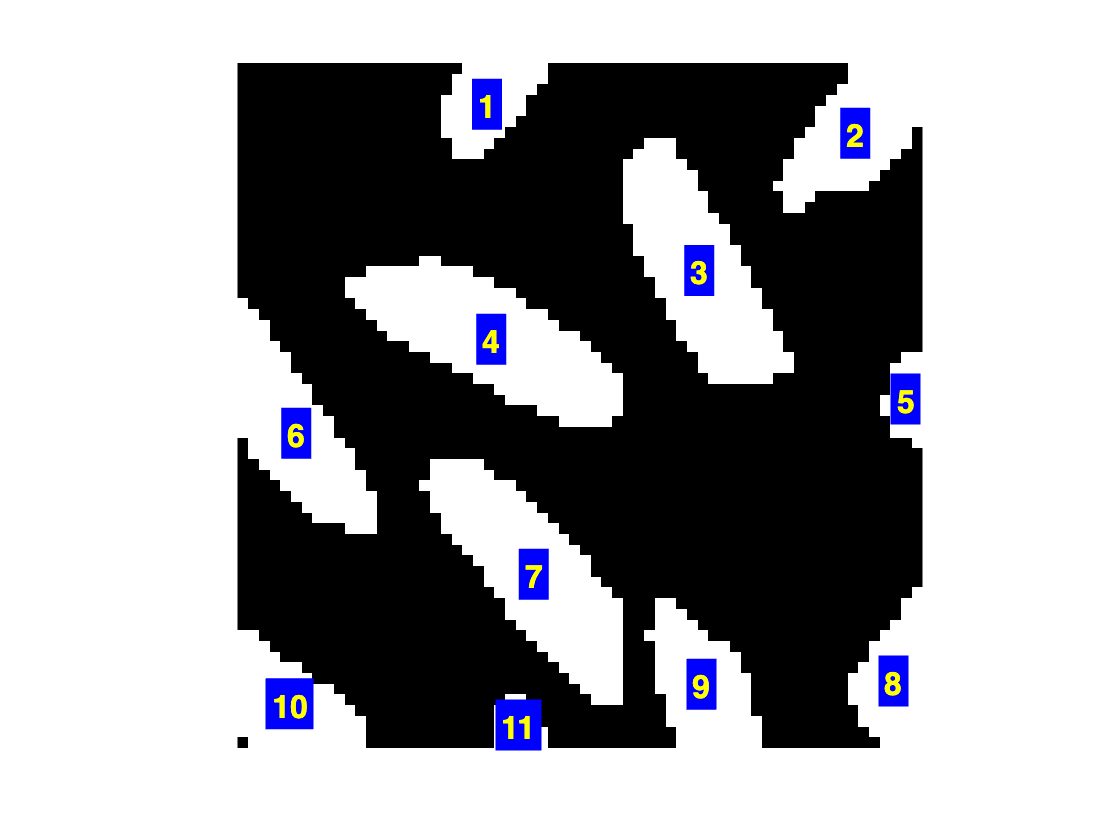For another example, you could sort by area.
props_by_area = sortrows(props,"Area","descend")
props_by_area = 11×4 table
AreaCentroidCentroid_xCentroid_y
120824.197126.312524.197126.3125
219928.185948.256328.185948.2563
318943.634919.878343.634919.8783
41385.985535.08705.985535.0870
511558.20877.069658.20877.0696
69743.845458.536143.845458.5361
7875.367860.35635.367860.3563
87061.785758.228661.785758.2286
96123.81974.344323.81974.3443
102662.923131.884662.923131.8846
112026.750062.350026.750062.3500
imshow(A)
for k = 1:height(props_by_area)
x = props_by_area.Centroid_x(k);
y = props_by_area.Centroid_y(k);
text(x,y,string(k),Color = "yellow", BackgroundColor = "blue", FontSize = 16, ...
FontWeight = "bold", HorizontalAlignment = "center", ...
VerticalAlignment = "middle");
end
title("Objects sorted by area (biggest to smallest)")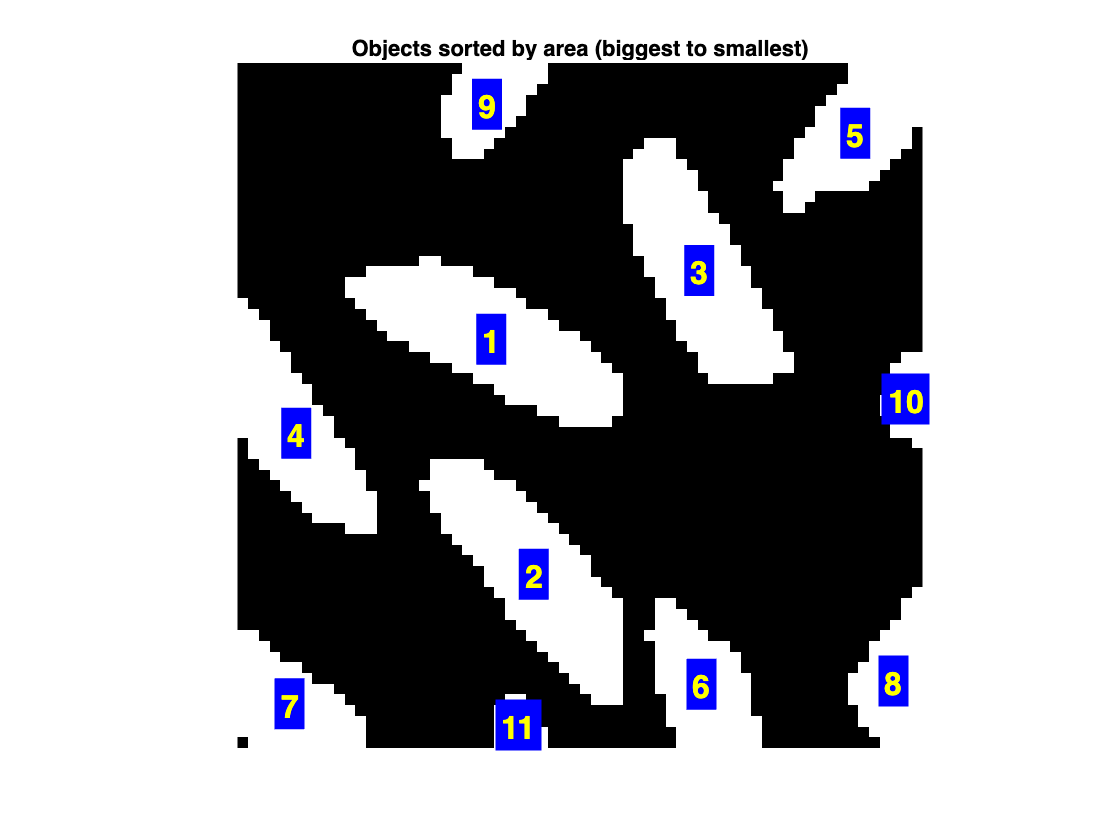|